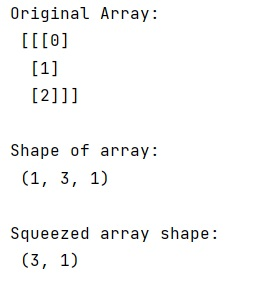# numpy.squeeze() Method | Why do we need numpy.squeeze()?

Learn about the numpy.squeeze() method and why do we need this method?
Submitted by Pranit Sharma, on February 21, 2023

NumPy is an abbreviated form of Numerical Python. It is used for different types of scientific operations in python. Numpy is a vast library in python which is used for almost every kind of scientific or mathematical operation. It is itself an array which is a collection of various methods and functions for processing the arrays.

## numpy.squeeze() Method

The numpy.squeeze() is used to remove axes of length one from an array. It takes an input array (arr) and an axis parameter. It selects a subset of the entries of length one in the shape. If an axis is selected with a shape entry greater than one, an error is raised.

It returns the same input array as an output but with all or a subset of the dimensions of length 1 removed. This is always arr itself or a view into arr. An important point is that if all axes are squeezed, the result is a 0d array and not a scalar.

Let us understand with the help of an example,

## Python code to demonstrate that why do we need numpy.squeeze()

```# Import numpy
import numpy as np

# Creating a numpy array
arr = np.array([[, , ]])

# Display original array
print("Original Array:\n",arr,"\n")

# First look at the shape of the array
print("Shape of array:\n",arr.shape,"\n")

# Removing an axes of length one using squeeze
res = np.squeeze(arr, axis=0).shape

# Display result
print("Squeezed array shape:\n",res,"\n")
```

Output: